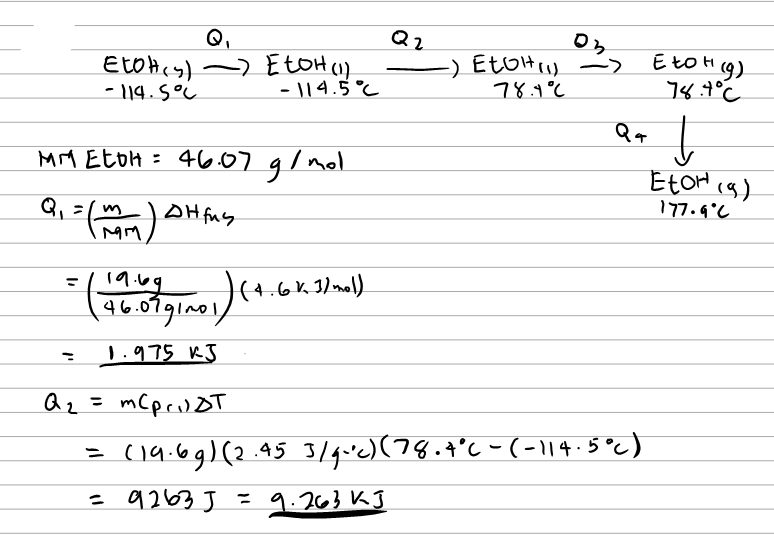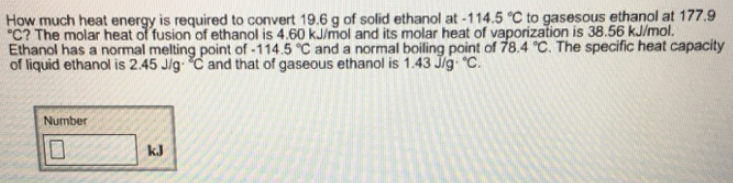# Problem: How much heat energy is required to convert 19.6 g of solid ethanol at-114.5°C to gasesous ethanol at 177.9°C? The molar heat of fusion of ethanol is 4.60 kJ/mol and its molar heat of vaporization is 38.56 kJ/mol. Ethanol has a normal melting point of-114.5°C and a normal boiling point of 78.4°C. The specific heat capacity of liquid ethanol is 2.45 J/g•°C and that of gaseous ethanol is 1.43 J/g·°C.

###### FREE Expert Solution100% (77 ratings)###### Problem Details

How much heat energy is required to convert 19.6 g of solid ethanol at-114.5°C to gasesous ethanol at 177.9°C? The molar heat of fusion of ethanol is 4.60 kJ/mol and its molar heat of vaporization is 38.56 kJ/mol. Ethanol has a normal melting point of-114.5°C and a normal boiling point of 78.4°C. The specific heat capacity of liquid ethanol is 2.45 J/g•°C and that of gaseous ethanol is 1.43 J/g·°C.